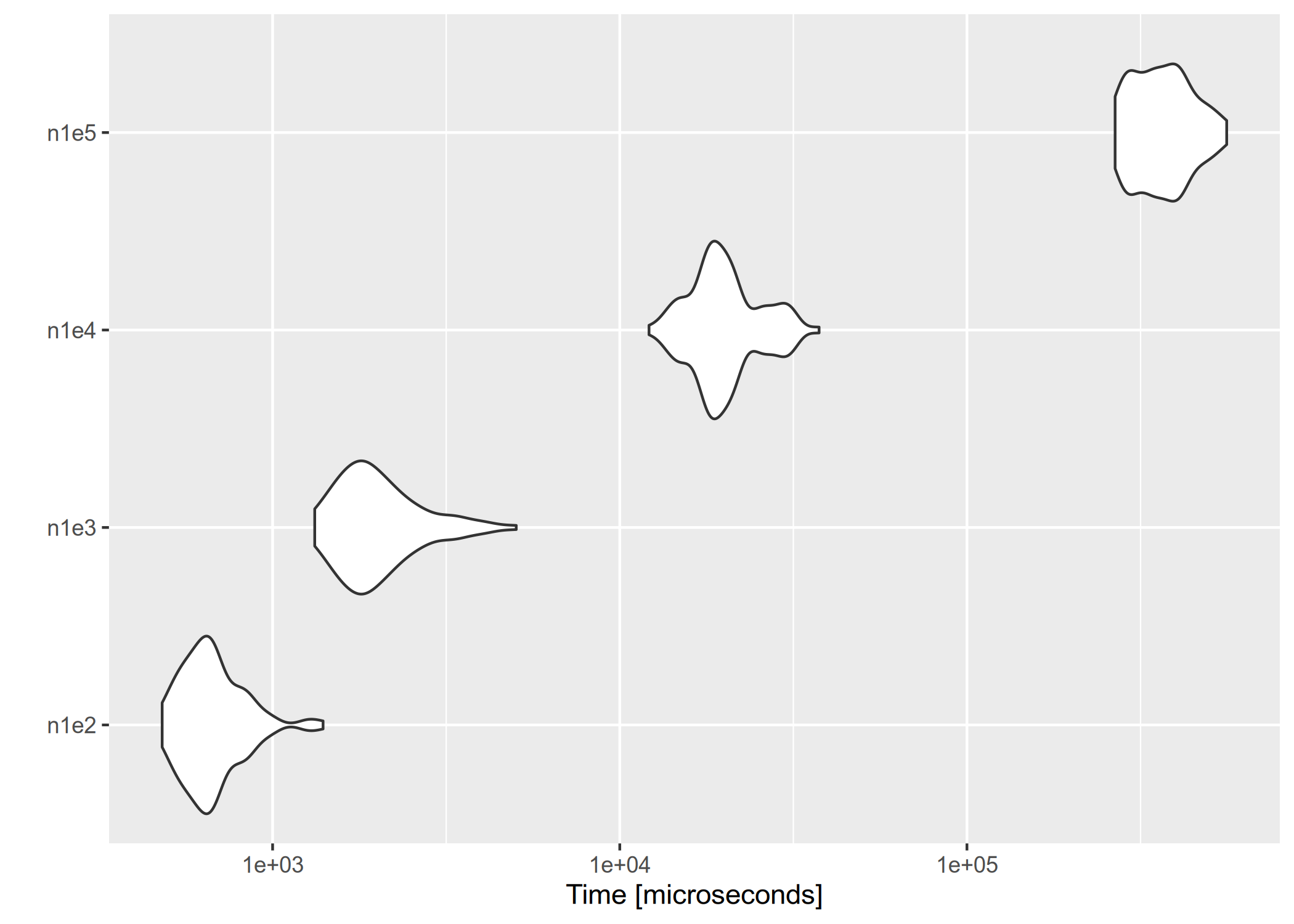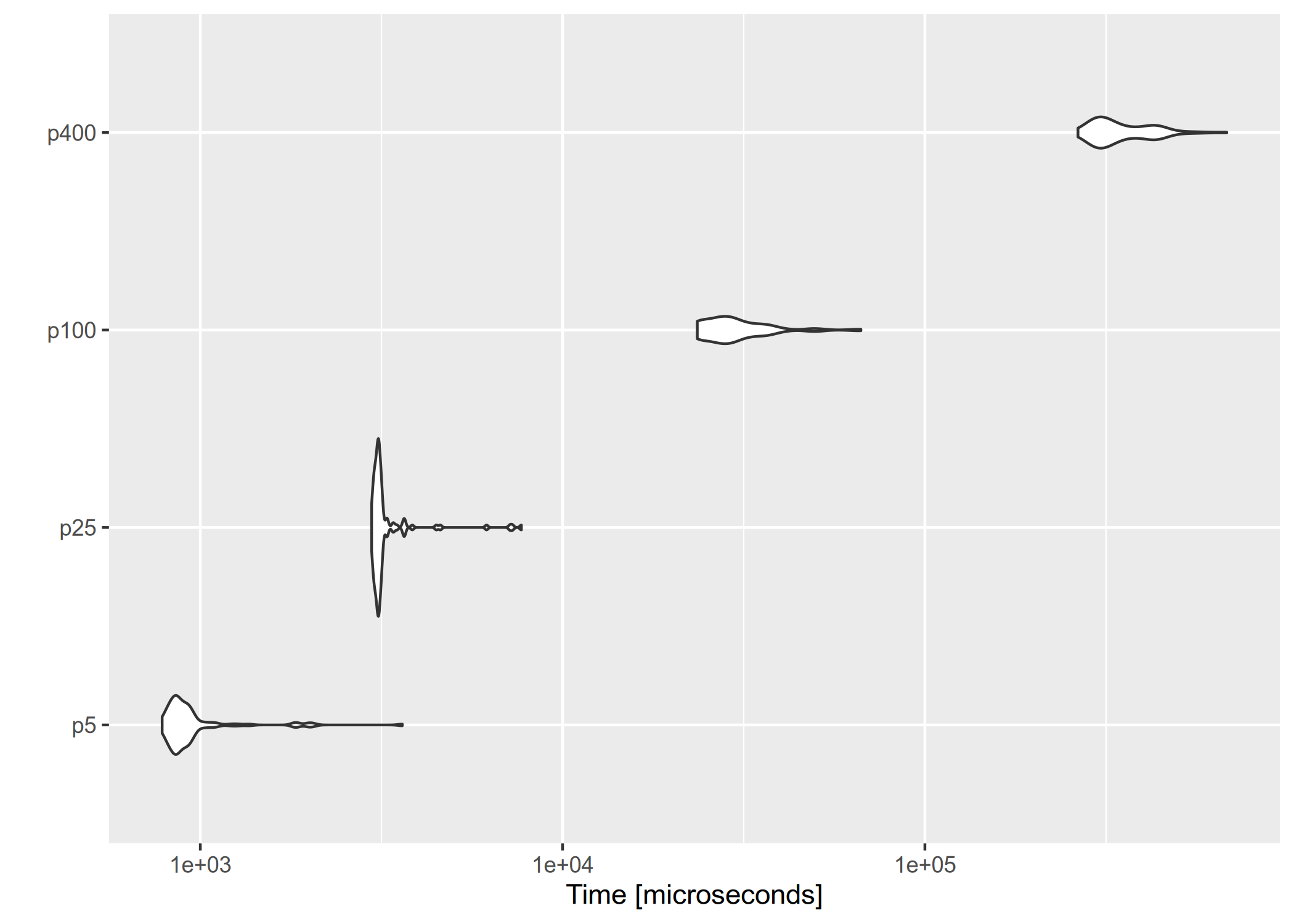# Performance

All the tests were done on an Arch Linux x86_64 machine with an Intel(R) Core(TM) i7 CPU (1.90GHz). We first load the necessary packages.

library(melt)
library(microbenchmark)
library(ggplot2)

## Empirical likelihood computation

We show the performance of computing empirical likelihood with el_mean(). We test the computation speed with simulated data sets in two different settings: 1) the number of observations increases with the number of parameters fixed, and 2) the number of parameters increases with the number of observations fixed.

### Increasing the number of observations

We fix the number of parameters at $$p = 10$$, and simulate the parameter value and $$n \times p$$ matrices using rnorm(). In order to ensure convergence with a large $$n$$, we set a large threshold value using el_control().

set.seed(3175775)
p <- 10
par <- rnorm(p, sd = 0.1)
ctrl <- el_control(th = 1e+10)
result <- microbenchmark(
n1e2 = el_mean(matrix(rnorm(100 * p), ncol = p), par = par, control = ctrl),
n1e3 = el_mean(matrix(rnorm(1000 * p), ncol = p), par = par, control = ctrl),
n1e4 = el_mean(matrix(rnorm(10000 * p), ncol = p), par = par, control = ctrl),
n1e5 = el_mean(matrix(rnorm(100000 * p), ncol = p), par = par, control = ctrl)
)

Below are the results:

result
#> Unit: microseconds
#>  expr        min          lq        mean     median          uq        max
#>  n1e2    480.702    579.4605    683.9713    651.999    744.1055   1397.514
#>  n1e3   1322.316   1639.4090   2089.1867   1879.296   2298.9635   5032.287
#>  n1e4  12140.334  17463.6515  20520.6089  19397.234  21753.5280  37504.643
#>  n1e5 267149.481 307769.8205 370535.4057 358940.570 414239.7385 560010.771
#>  neval cld
#>    100 a
#>    100 a
#>    100  b
#>    100   c
autoplot(result)### Increasing the number of parameters

This time we fix the number of observations at $$n = 1000$$, and evaluate empirical likelihood at zero vectors of different sizes.

n <- 1000
result2 <- microbenchmark(
p5 = el_mean(matrix(rnorm(n * 5), ncol = 5),
par = rep(0, 5),
control = ctrl
),
p25 = el_mean(matrix(rnorm(n * 25), ncol = 25),
par = rep(0, 25),
control = ctrl
),
p100 = el_mean(matrix(rnorm(n * 100), ncol = 100),
par = rep(0, 100),
control = ctrl
),
p400 = el_mean(matrix(rnorm(n * 400), ncol = 400),
par = rep(0, 400),
control = ctrl
)
)
result2
#> Unit: microseconds
#>  expr        min          lq        mean     median         uq        max neval
#>    p5    784.742    843.6425    987.3792    880.003    940.296   3610.111   100
#>   p25   2971.194   3048.8325   3320.6049   3105.648   3163.769   7695.062   100
#>  p100  23538.566  26370.0610  30707.9708  29054.183  32858.164  66575.924   100
#>  p400 264627.376 297454.1460 354283.8642 321911.429 407738.503 680908.163   100
#>  cld
#>  a
#>  a
#>   b
#>    c
autoplot(result2)On average, evaluating empirical likelihood with a 100000×10 or 1000×400 matrix at a parameter value satisfying the convex hull constraint takes less than a second.